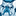Announcements
You can succeed best and quickest by helping others to succeed. Join the conversation.
cancel
Showing results for
Did you mean:Contributor III

## Handling field names with spaces in ODAG script

Good day

I am trying to build an On Demand app using the script below in template app to bind. My problem is that there are certain field names with spaces such as "Customer ID " in script below.The code shows syntax error on all instances where "Customer ID" is included. Unfortunately I cannot change the field names because these fields are used in other systems which would also need to be changed. Is there any way I can go around this problem? I have tried using "", ``, ' ' but none have worked.

//Creates a WHERE statement which can be used in table loads to restrict the data to a smaller set

SUB ExtendWhere(Name, ValVarName)
LET T = Name & '_COLNAME';
LET ColName = \$(T);
LET Values = \$(ValVarName);
IF len(Values) > 0 THEN
IF len(WHERE_PART) > 0 THEN //If WHERE clause exists then extend it with further values
LET WHERE_PART = '\$(WHERE_PART) AND MATCH(\$(ColName),\$(Values))';
ELSE
LET WHERE_PART = ' WHERE MATCH(\$(ColName),\$(Values))'; //If WHERE clause does not exist then create it
ENDIF
ENDIF
END SUB;

////////////////////////////////////////////////////////////////////////////////////////////////////////////////////////////////////////////////////////////////////////////

//Here we set the variables ready to hold the names of any fields we want considering for ODAG filtering (selection)
//The ods_ field names bind the fields to those in your Selections Application.

SET Sel_Implementation = ; //resets the variable from any previous reload
SET Sel_Implementation = \$(od_Implementation); // follow 'ods_' with the field name as it appears in your UI / final load
SET Sel_Implementation_COLNAME ='Implementation'; // enter the field name as it appears in the source data

SET "Sel_Customer ID" = ;
SET "Sel_Customer ID"= \$(od_Customer ID);
SET "Sel_Customer ID_COLNAME" ='Customer ID';
///////////////////////////////////////////////////////////////////////////////////////////////////////////////////////////////////////////////////////////////////////
//This section builds the WHERE_PART variables that are passed to the SUB

SET WHERE_PART = '';
// WHERE_PART to be applied to only TIM loads that contain Suffix
FOR EACH fldname IN 'Sel_Implementation', 'Sel_Customer ID'
LET vallist = \$(fldname);
WHEN (IsNull(vallist)) LET vallist = '';
IF len(vallist) > 0 THEN
CALL ExtendWhere('\$(fldname)','\$(vallist)');
ENDIF
NEXT fldname

TRACE Generated WHERE clause: ;
TRACE \$(WHERE_PART);

1 Solution

Accepted SolutionsMVP

This is what I have (anonymized my field name)

``````SUB ExtendWhere(Name, ValVarName)
LET T = Name & '_COLNAME';
LET ColName = \$(T);
LET Values = \$(ValVarName);
IF len(Values) > 0 THEN
IF len(WHERE_PART) > 0 THEN
LET WHERE_PART = '\$(WHERE_PART) and Match([\$(ColName)], \$(Values))';
ELSE
LET WHERE_PART = 'Where Match([\$(ColName)], \$(Values))';
ENDIF
ENDIF
END SUB;``````

and then this

``````SET PT = ;
SET PT = \$(ods_P T);
SET PT_COLNAME = 'P T';``````

and then this

``````FOR EACH fldname IN 'PT'
LET vallist = \$(fldname);
WHEN (IsNull(vallist)) LET vallist = '';
IF len(vallist) > 0 THEN
CALL ExtendWhere('\$(fldname)','\$(vallist)');
ENDIF
NEXT fldname``````

11 RepliesMVP

Can you see if this makes any difference (adding square brackets around the field name)

SET "Sel_Customer ID" = ;
SET "Sel_Customer ID"= \$(od_Customer ID);
SET "Sel_Customer ID_COLNAME" ='[Customer ID]';Contributor III
Author

@sunny_talwar  adding square brackets fixes the syntax error but I get an "UNexpected token" error when I try to generate an appMVPContributor III
Author

No the load fails almost instantly with no other progress message. Please check attached imageMVP

Okay, so I think I have done this in one my scripts

SUB ExtendWhere(Name, ValVarName)
LET T = Name & '_COLNAME';
LET ColName = \$(T);
LET Values = \$(ValVarName);
IF len(Values) > 0 THEN
IF len(WHERE_PART) > 0 THEN //If WHERE clause exists then extend it with further values
LET WHERE_PART = '\$(WHERE_PART) AND MATCH([\$(ColName)],\$(Values))';
ELSE
LET WHERE_PART = ' WHERE MATCH([\$(ColName)],\$(Values))'; //If WHERE clause does not exist then create it
ENDIF
ENDIF
END SUB;

and change the previous back to how you had it initially (with no square brackets)

SET "Sel_Customer ID" = ;
SET "Sel_Customer ID"= \$(od_Customer ID);
SET "Sel_Customer ID_COLNAME" ='Customer ID';Contributor III
Author

Unfortunately that did not work. I also tried " " in place of the square brackets. Could you possibly send a section of your code where you applied this technique?MVP

This is what I have (anonymized my field name)

``````SUB ExtendWhere(Name, ValVarName)
LET T = Name & '_COLNAME';
LET ColName = \$(T);
LET Values = \$(ValVarName);
IF len(Values) > 0 THEN
IF len(WHERE_PART) > 0 THEN
LET WHERE_PART = '\$(WHERE_PART) and Match([\$(ColName)], \$(Values))';
ELSE
LET WHERE_PART = 'Where Match([\$(ColName)], \$(Values))';
ENDIF
ENDIF
END SUB;``````

and then this

``````SET PT = ;
SET PT = \$(ods_P T);
SET PT_COLNAME = 'P T';``````

and then this

``````FOR EACH fldname IN 'PT'
LET vallist = \$(fldname);
WHEN (IsNull(vallist)) LET vallist = '';
IF len(vallist) > 0 THEN
CALL ExtendWhere('\$(fldname)','\$(vallist)');
ENDIF
NEXT fldname``````Contributor III
Author

@sunny_talwar  Thanks alot.That helpedMVP

What exactly was the issue? What did you change?Tags
Community Browser